# Smoother Switching Between Pumps in SWMM 5 – A better simulation of a VSP?

SubjectSmoother Switching Between Pumps in SWMM 5 – A better simulation of a VSP?
An oft requested feature in SWMM 5 is the ability to better simulate a variable speed pump.   The basic feature we are trying to model is multiple pumps between two nodes, one pump curve for all of the pumps and the ability to turn on and turn off the pumps based on either the head or depth at a Wet Well (Figure 1).  You can turn on or off the pumps Pump1, Pump2 and Pump3 based on the depth at the Wet Well but this feature is stepwise linear and usually uses three pump curves.  A better way to simulate this feature is to use the SWMM 5 Real Time Rules (RTC) to simulate the Pump setting based on a control curve.
The Pump flow at any time step is the Pump Flow estimated from the Pump Curve (Figure 2) * The Pump Setting (Figure 3)
Each of the three pumps has a different Control Curve (Figure’s 4, 5 and 6, respectively) which turns on or turns off the Pump based on a range of Wet Well Depths.  The overall effect is that the total flow summing all three pumps together is smoother (Figure 7 and Figure 8) and the user can simulate different pump speeds based on the same pump curve depending on which pump is currently on.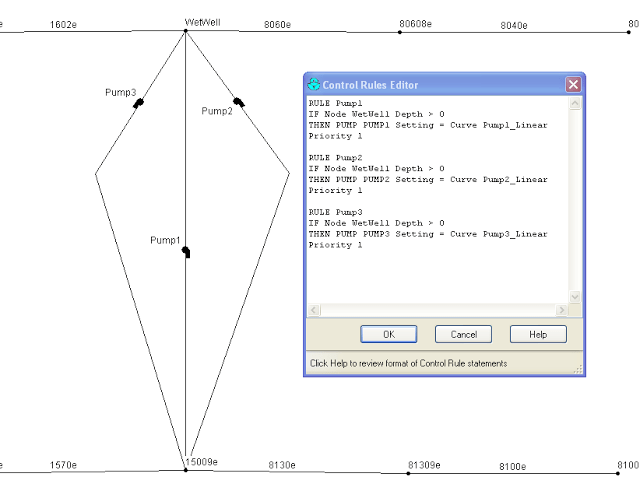Figure 1.   Example RTC Rules and VSP Pumps in a SWMM 5 model.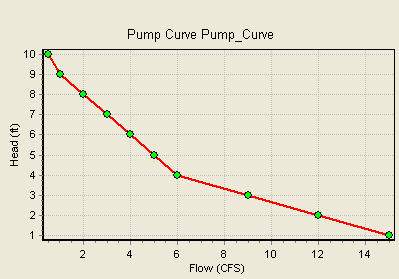Figure 2.  The Pump Curve Used for all 3 Pumps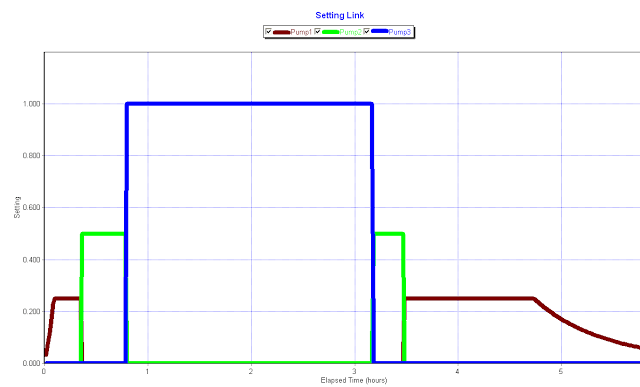Figure 3.  The Pump Setting for all Three Pumps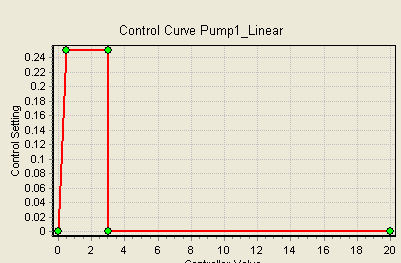Figure 4.   Pump Control Curve for Pump 1.  The Pump has a Setting of ¼ between 0.5 and 3 feet at the node Wet Well and zero otherwise.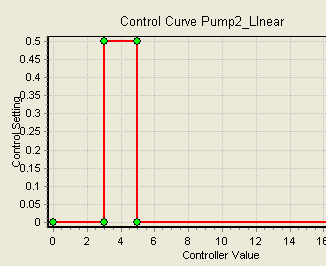Figure 5.   Pump Control Curve for Pump 2.  The Pump has a Setting of 1/2 between 3 and 5 feet at the node Wet Well and zero otherwise.Figure 6.   Pump Control Curve for Pump 3.  The Pump has a Setting of 1 above 5 feet at the node Wet Well and zero otherwise.Figure 7.  The Flow in all 3 Pumps.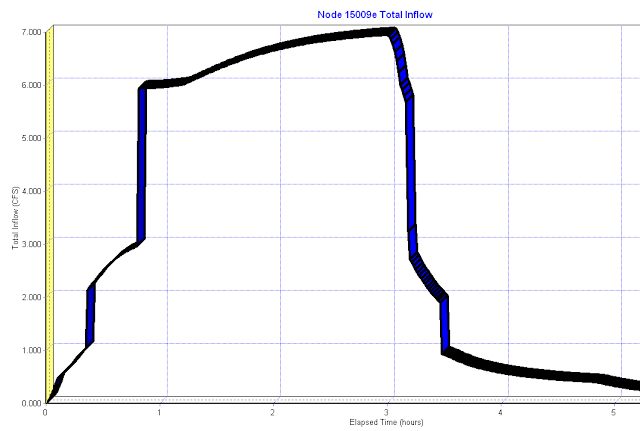Figure 8.  The total flow from all three Pumps to the downstream node.

Translate »